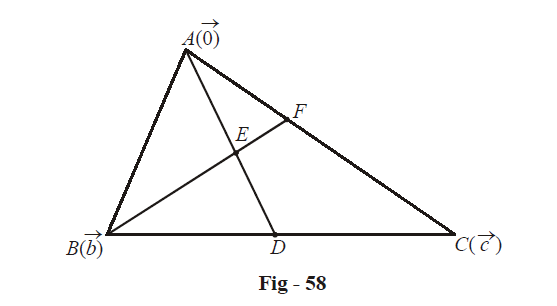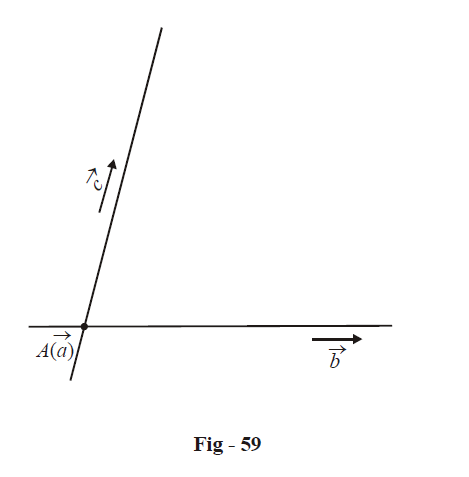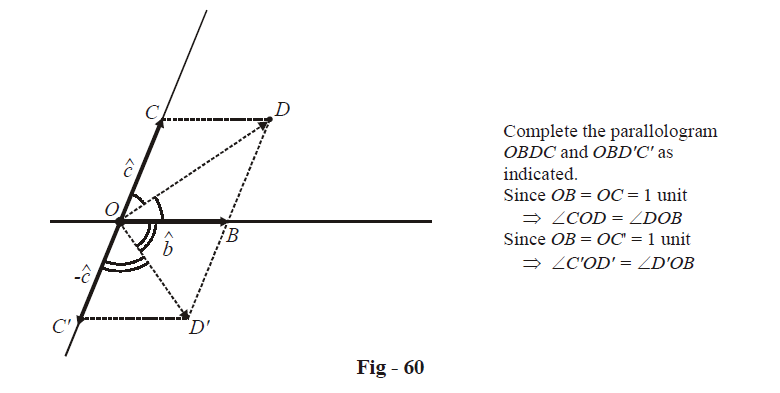# Examples On Vector Equations Of Lines Set-2﻿

Go back to  'Vectors and 3-D Geometry'

Example – 46

The median AD of a triangle ABC is bisected at E and BE is produced to meet AC in F. Prove by vector methods that \begin{align}EF=\frac{1}{4}BF.\end{align}

Solution:Assume A to be the origin and B, C to be the points  $$\vec{b},\,\,\vec{c}$$ respectively. We have,

\begin{align}&\quad\quad \;\;\,D\equiv \frac{\vec{b}+\vec{c}}{2} \\ & \Rightarrow \quad E\equiv \frac{\vec{b}+\vec{c}}{4} \\ & \Rightarrow \quad \text{ }Equation\text{ }of\,BE:\,\,\vec{r}=\vec{b}+\lambda \left( \frac{\vec{b}+\vec{c}}{4}-\vec{b} \right) \\ & \Rightarrow \quad \vec{r}=\left( 1-\frac{3\lambda }{4} \right)\vec{b}+\frac{\lambda }{4}\vec{c} \\ \end{align}

Also, $$Equation\text{ }of\,AC:\vec{r}={\lambda }'\vec{c}$$

Since AC and BE intersect in F, there must be some $$\lambda ,\,{\lambda }'\in \mathbb{R}$$ such that

\begin{align}& \left( 1-\frac{3\lambda }{4} \right)\vec{b}+\frac{\lambda }{4}\vec{c}={\lambda }'\vec{c} \\\\ & \Rightarrow \quad \lambda =\frac{4}{3},\,\,{\lambda }'=\frac{1}{3} \\\\ & \Rightarrow\quad F\equiv \frac{{\vec{c}}}{3} \\ \end{align}

If E divides BF in the ratio k : 1, we have

\begin{align} & \frac{k\left( \frac{{\vec{c}}}{3} \right)+1\left( {\vec{b}} \right)}{k+1}=\frac{\vec{b}+\vec{c}}{4} \\\\ & \Rightarrow \quad 4k\vec{c}+12\vec{b}=3\left( k+1 \right)\vec{b}+3\left( k+1 \right)\vec{c} \\ \\ & \Rightarrow \quad k=3 \\ \end{align}

Thus,

$BE:EF=3:1\Rightarrow \qquad EF:BF=1:4$

Example – 47

Find the equation of the bisector(s) of the angle(s) between the straight lines  $$\vec{r}=\vec{a}+\lambda \vec{b}\,\,and\,\,\vec{r}=\vec{a}+\mu \vec{c}.$$

Solution: Note that the lines pass through $$A(\vec{a})$$ and are respectively parallel to $$\vec{b}\,\,and\,\,\vec{c}:$$We need to find the vector(s) equally inclined to $$\vec{b}\,\,and\,\,\vec{c}$$ to be able to write the equation (s) of the angle bisector(s). For this purpose, consider the co-initial unit vectors $$\hat{b}\,\,and\,\,\hat{c}:$$It should be clear from this figure that the two vectors equally inclined to $$\hat{b}\,\,and\,\,\hat{c}$$are simply the diagonals of the two parallelograms drawn, i.e., $$\overrightarrow{OD}\,\,and\;\overrightarrow{OD'}.$$ Thus, the directions of the two angle bisectors are given by the vectors $$(\hat{b}+\hat{c})\,\,and\,\,(\hat{b}-\hat{c}).$$

Since the angle bisectors we require both pass through $$\vec{a},$$ we have their equations as

\begin{align}&\quad\qquad\;\;\,\vec{r}=\vec{a}+\lambda(\hat{b}+\hat{c})\,\,and\,\,\vec{r}=\vec{a}+\lambda \left( \hat{b}-\hat{c} \right)\qquad\quad\quad,\lambda \in \mathbb{R} \\ & \Rightarrow \qquad \vec{r}=\hat{a}+\lambda \left\{ \frac{{\vec{b}}}{\left| {\vec{b}} \right|}\pm \frac{{\vec{c}}}{\left| {\vec{c}} \right|} \right\}\qquad\qquad\qquad,\lambda \in \mathbb{R} \\ \end{align}

Vectors
grade 11 | Questions Set 1
Vectors